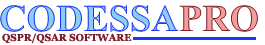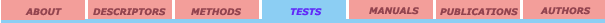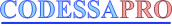External validation set Leave-One-Out crossvalidation Leave-Many-Out crossvalidation Randomization test

## Randomization test

Randomization tests  are statistical tests in which the data are repeatedly elaborated; a test statistic (r2, t-criteria, F-criteria, etc.) is computed for each data permutation and the proportion of the data permutations, with test statistics values as large as the value for the obtained results, determines the significance of the results. For the testing of the multilinear correlation, the vector Y permutations are processed through multilinear regression procedures with fixed columns of matrix X. Due to a factorial increase in time spent from the size of the vector Y, Monte-Carlo method is often be used for producing randomization test.

References:

1. Edington E. S. Randomization tests: Marsel Dekker, Inc.: New York and Basel, 1980, pp.195-216.University of Florida 2001. All rights reserved